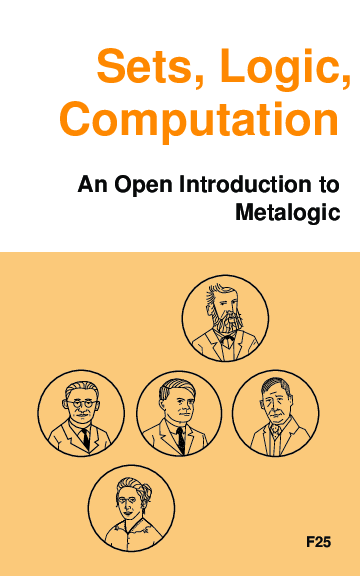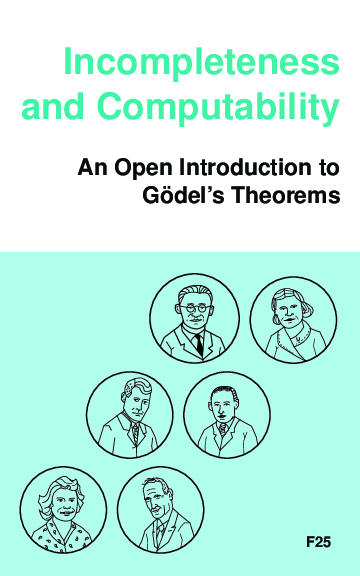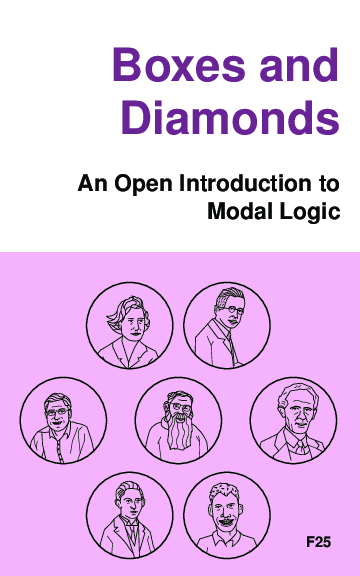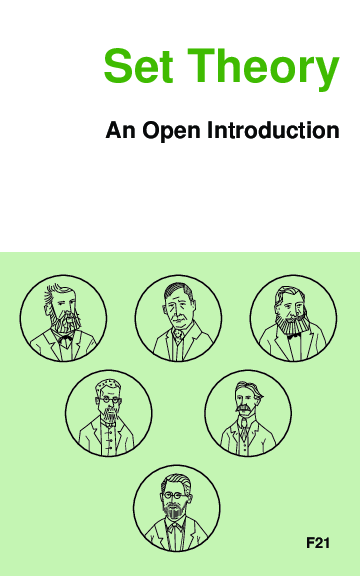Open Logic Project Builds Site

Open Logic Project Builds

This site contains PDFs built from the source LaTeX files of the most recent version of the Open Logic Text.

Complete PDFs

We have PDFs of the complete text in the Open Logic master branch, arranged in a somewhat sensible manner, including editorial comments. It's not intended as a textbook, but it shows what's there.

Individual Textbooks

There are already a few textbooks that show how one might "remix" the material in the Open Logic Project to produce nice textbooks. These vary in the material that's included, the design, and the configuration options used. They may also include additional material specific to those courses.

Sets, Logic, ComputationA textbook on metalogic, developed for Calgary's Logic II course: includes the material on set theory, first-order logic (including both sequent calculus and natural deduction), and Turing machines (including undecidability). Appendices cover proofs and induction and biographies of some logicians. This text also includes chapter summaries and a glossary. Problems are collected at the end of chapters.

• slc-screen for screen reading (color, smaller margins, low-res images)
• slc-print for print-on-demand (without cover)
• Source code for generating this textbook is on GitHub in a separate repository.

Incompleteness and ComputabilityA textbook on Gödel's incompleteness theorems and computability theory, developed for Calgary's Logic III course: includes the material on recursive functions, Gödel's incompleteness theorem, models of arithmetic, second-order logic, and the lambda calculus. Appendices cover basics of first-order logic with natural deduction, and biographies of some logicians. This text also includes chapter summaries.

• ic-screen for screen reading (color, smaller margins, low-res images)
• ic-print for print-on-demand (without cover)
• Source code for generating this textbook is on GitHub in a separate repository.

Boxes and DiamondsA textbook for modal and other intensional logics based on the Open Logic Project; includes the material on normal modal logic, intutionistic logic, and counterfactuals, with appendices on basic set theory and classical propositional logic.

• bd-screen for screen reading (color, smaller margins, low-res images)
• Source code for generating this textbook is on GitHub in a separate repository.

Set Theory: An Open IntroductionA brief introduction to the philosophy of set theory; covers why set theory came about; how to reduce large swathes of mathematics to set theory + arithmetic; how to embed arithmetic in set theory; what the cumulative iterative conception of set amounts to; how one might try to justify the axioms of ZFC. Most of the material making up the text comes from Tim Button's Open Set Theory.

• settheory-screen for screen reading (color, smaller margins, low-res images)
• Source code for generating this textbook is on GitHub in a separate repository.

Intermediate Logic

The textbook used for McGill's Intermediate Logic course: includes material on set theory, first-order logic, completeness and incompleteness theorems. It was the first remix of the OLT and hasn't been used since 2015, but the content updates with the main repository. This one collects problems at the end.

Sample Texts

The OLP main repository also includes two sample textbooks that serve just to illustrate how one might produce texts using different formatting conventions.

• open-logic-enderton, a sample textbook that follows the conventions of Enderton's popular A Mathematical Introduction to Logic.
• open-logic-sample, a selection including set theory, first-order logic, Turing machines, and incompleteness, using yet other conventions.

Active Branches

At any point, there may be active branches of the Open Logic Project in GitHub. This branches may contain additional material, or revisions of the material in the master branch which are being worked on or reviewed for inclusion. We provide PDFs of the complete text as it looks in these branches.